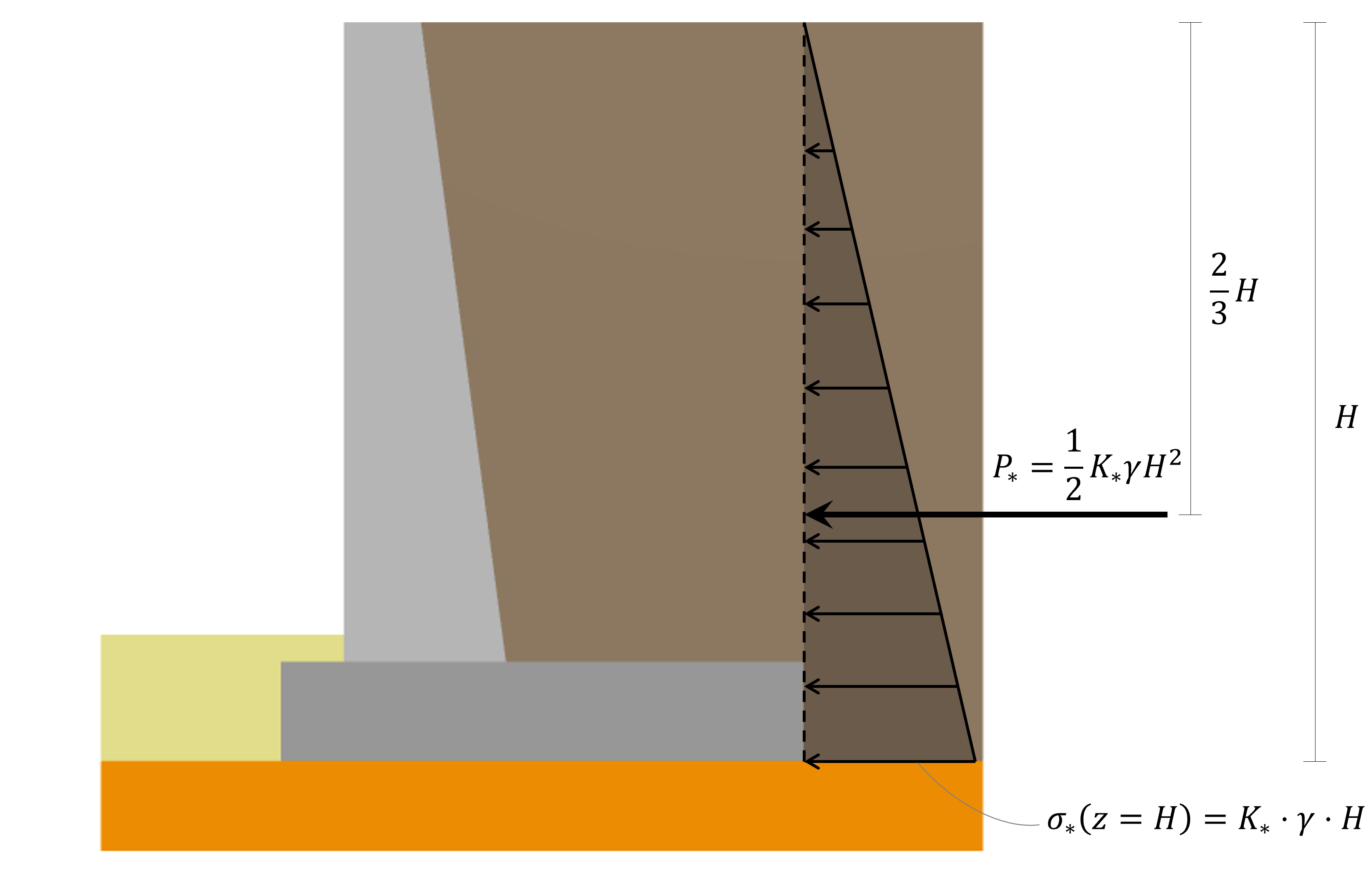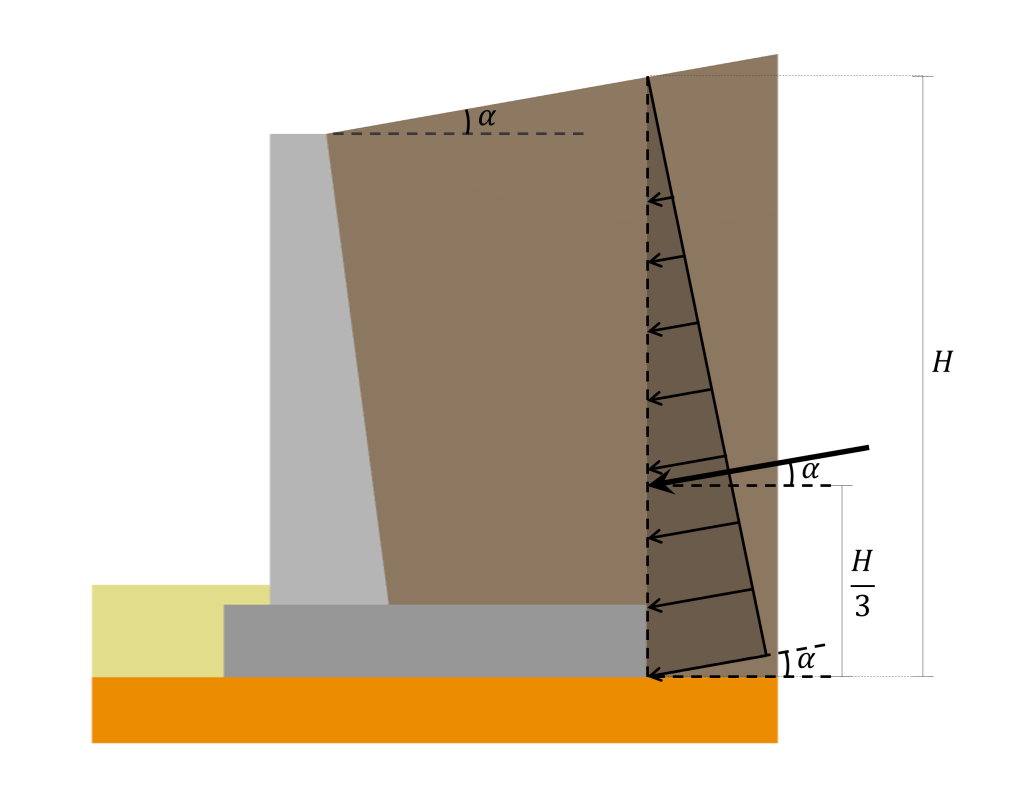Documentação SkyCiv

Seu guia para o software SkyCiv - tutoriais, guias de instruções e artigos técnicos

1. Casa
2. Muro de Contenção SkyCiv
3. Artigos e tutoriais
4. Pressão de Terra Lateral para Projeto de Muro de Retenção

# Calculating Lateral Earth Pressure on a Retaining Wall

One of the main loads acting on a retaining wall is the lateral earth pressure. Cálculo da pressão lateral de terra em um muro de arrimo Uma das principais cargas que atuam em um muro de arrimo é a pressão lateral de terra, doing a good estimation of its magnitude and distribution is decisive in the design of a Concrete Retaining Wall. No geral, there are three different types of lateral earth pressure depending on the direction that the wall tends to move:

• Cálculo da pressão lateral de terra em um muro de arrimo Uma das principais cargas que atuam em um muro de arrimo é a pressão lateral de terra: When the wall is completely restrained from moving
• Active earth pressure: When the wall may tilt away from the retained soil
• Pressão passiva de terra: When the wall may be pushed into the retained soil

Neste artigo, we will focus on describing the formulas for each of the earth pressure cases mentioned above.

## Lateral earth pressure distribution

No geral, Formulas e equações do momento flector. Having a zero value at the surface and a maximum value at the deepest point following a linear distribution between the two mentioned boundaries. Portanto, the horizontal subsurface stress distribution is described by the following expression:

$$\sigma_h = K_* \cdot (\gamma z)$$

Onde $$K_*$$ takes the value of $$K_o$$ for the case of at-rest pressure, $$K_a$$ for the case of active pressure, e $$K_p$$ for the case of passive pressure.

Integrating the given expression for the lateral subsurface stress from $$0$$ para $$H$$ no $$[object Window]), the resultant turns out to be: \(P_*=\frac{1}{2} K_* \cdot \gamma \cdot H^2$$

This resultant’s line of action is located $$\fratura{2}{3}H$$ from the surface. The lateral earth pressure distribution, its resultant, and location described above are illustrated in the following picture:It is important to mention that the presented distribution and resultant calculation approach only applies to soil pressures acting on a vertical backface. In the case of a retaining wall with an inclined backface (like the one in the picture above), the surface where the soil pressure acts is still considered vertical since it is assumed to act in a vertical plane located where the heel ends.

Além disso, when the backfill is inclined at some angle $$\alpha$$ with respect to the horizontal, the pressure distribution and its resultant are inclined at that same angle $$\alpha$$ as illustrated in the following:Correctly estimating the lateral earth pressure distribution and its resultant is a crucial step in the Retaining Wall Design Process. = H, Para obter mais informações sobre como esta pressão lateral de terra é incluída no Muro de Contenção aqui. Let’s now dive into the formulas for calculating the resultant lateral earth pressure exerted on a Retaining Wall by the soil in different conditions.

## At-rest lateral earth pressure

This approach for calculating the lateral earth pressure against a Retaining Wall can only be used if the wall is completely at rest and is not allowed to move either away from the soil or into the soil, this condition ensures that the horizontal strain in the soil is zero. a distribuição de pressão inclina um ângulo igual à inclinação do aterro, the coefficient of at-rest pressure ($$K_o$$) is the one that replaces $$K_*$$ in the previous equations. That coefficient is the only unknown for calculating the pressure distribution and its resultant. For normally consolidated soil, the relation for $$K_o$$ é:

$$K_o = 1-sin(\De acordo com Meyerhof)$$

Onde $$\[object Window]) is the effective angle of friction of the soil in consideration. For overconsolidated soil, the coefficient may be calculated using the following expression: \(K_o = (1-sem(\De acordo com Meyerhof))\cdot OCR^{sem(\De acordo com Meyerhof)}$$

Onde $$\[object Window]) is the effective angle of friction, e \(OCR$$ the overconsolidation ratio of the soil in consideration.

Plugging this coefficient into the expression for calculating the resultant force from the lateral earth pressure at-rest yields to:

$$P_o=\frac{1}{2} \gamma \cdot H^2 \cdot K_o$$

## Active lateral earth pressure

The previous approach can be used when the wall does not yield at all, Contudo, if a wall tends to move away from the soil, the soil pressure on the wall at any depth will decrease. a distribuição de pressão inclina um ângulo igual à inclinação do aterro, the coefficient of at-rest pressure ($$K_a$$) is the one that replaces $$K_*$$ in the initial equations. Using Rankine’s approach for a granular backfill, and assuming that the pressure acts in a vertical backface, the active earth-pressure coefficient may be calculated using the equation:

$$K_a=cos(\alpha) \fratura{cos(\alpha) – \sqrt{cos^2(\alpha) – cos^2(\De acordo com Meyerhof)}}{cos(\alpha) + \sqrt{cos^2(\alpha) – cos^2(\De acordo com Meyerhof)}}$$

Onde $$\[object Window]) is the angle of friction of the soil in consideration and \(\alpha$$ is the angle of inclination of the backfill surface with respect to the horizontal.

Plugging this coefficient into the expression for calculating the resultant force from the lateral earth pressure at active condition yields to:

$$P_a=\frac{1}{2} \gamma \cdot H^2 \cdot K_a$$

The Rankine active pressure calculations presented before are based on the assumption that the wall is frictionless.

## Passive lateral earth pressure

The lateral earth pressure acting on a retaining wall is considered passive when the wall is pushed into the soil mass, in that condition, the horizontal stress will increase with respect to the at-rest condition. a distribuição de pressão inclina um ângulo igual à inclinação do aterro, the coefficient of at-rest pressure ($$K_p$$) is the one that replaces $$K_*$$ in the initial equations. Using Rankine’s approach for a granular backfill, and assuming that the pressure acts in a vertical backface, the passive earth-pressure coefficient may be calculated using the expressions:

When the backfill is completely horizontal

$$K_p = tan^2(45º+\frac{\De acordo com Meyerhof}{2})$$

When the backfill is inclined a certain angle with respect to the horizontal

$$K_p=cos(\alpha) \fratura{cos(\alpha) + \sqrt{cos^2(\alpha) – cos^2(\De acordo com Meyerhof)}}{cos(\alpha) – \sqrt{cos^2(\alpha) – cos^2(\De acordo com Meyerhof)}}$$

Onde $$\[object Window]) is the angle of friction of the soil in consideration and \(\alpha$$ is the angle of inclination of the backfill surface with respect to the horizontal.

Plugging this coefficient into the expression for calculating the resultant force from the lateral earth pressure at passive condition yields to:

$$P_p=\frac{1}{2} \gamma \cdot H^2 \cdot K_p$$

Novamente, the Rankine active pressure calculations presented before are based on the assumption that the wall is frictionless.

## Referências

também é aplicável para calcular a capacidade de carga final de estacas em argila, B. M. (2010). também é aplicável para calcular a capacidade de carga final de estacas em argila, a pressão exercida na parede segue uma distribuição vertical uniforme. Capítulo 7 a pressão exercida na parede segue uma distribuição vertical uniforme. a pressão exercida na parede segue uma distribuição vertical uniforme.

## Calculadora do muro de arrimo

Para obter mais informações sobre como esta pressão lateral de terra é incluída no Muro de Contenção, Para obter mais informações sobre como esta pressão lateral de terra é incluída no Muro de Contenção. A SkyCiv oferece uma calculadora gratuita de muro de arrimo que verifica o deslizamento no muro de arrimo e realiza uma análise de estabilidade em seus muros de arrimo, A SkyCiv oferece uma calculadora gratuita de muro de arrimo que verifica o deslizamento no muro de arrimo e realiza uma análise de estabilidade em seus muros de arrimo, Para obter mais informações sobre como esta pressão lateral de terra é incluída no Muro de Contenção, deslizamento, Para obter mais informações sobre como esta pressão lateral de terra é incluída no Muro de Contenção!oscar sanches
Desenvolvedor de Produto
BEng (Civil)